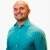# Learn how to use the one to one property of logarithms to help solve an equationThis math lesson will look at an example problem on logs to explain how to use the one to one property of logarithms to help solve an equation.
2 Views